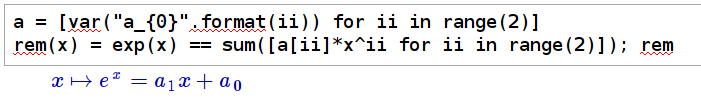## Sunday, 13 January 2013

### Cayley-Hamilton Theorem

My final post (so far) on matrix conjugation is the most useful of matrix theories and one of my personal favourites.

"In linear algebra, the Cayley–Hamilton theorem (named after the mathematicians Arthur Cayley and William Hamilton) states that every square matrix over a commutative ring (such as the real or complex field) satisfies its own characteristic equation."

This means that once you have the characteristic polynomial of a matrix M (for a 3x3 matrix in this example):
P(x) = a0 + a1x + a2x2 + x3= 0
then replacing x with M:
P(M) = a0I + a1M + a2M2 + M3= 0
will also be true.

Notice that we've replaced the constant term a0 with a0I, guaranteeing that this will give us a square matrix.
Let's prove this using conjugation. Basically every power of M can be replaced with a diagonal decomposition Mn = V Dn V-1:

P(M) = a0I + a1M + a2M2 + M3
P(M) = a0VD0V-1 + a1VD1V-1 + a2VD2V-1 + VD3V-1
Notice that we replaced I with a zero power of D. We can then collect the V and V-1 terms outside of the matrices Dn to get the following:
P(M) = V(a0D0+ a1D1+ a2VD2+ D3)V-1

Remembering that D consists of the eigenvalue solutions to the characteristic polynomial, and that powers of D are also diagonal powers of the eigenvalues, it should be clear that all matrix elements of P(M) inside V and V-1 will evaluate to 0, thus proving Cayley-Hamilton's theorem.

Why is this so useful? Well any polynomial Q(M) of order larger than n can be rewritten into a multiple of the characteristic polynomial P(M) and some remainder R(M).
Q(M) = p P(M) + R(M)
Where p is a positive integer and R(M) is a polynomial of order lower than n. Since P(M) = 0, any polynomial will reduce to R(M).This also works for infinite polynomials such as the Taylor expansions of sin, cos, exp, etc.

Let's read that again ... infinite polynomials over matrices can be reduced to a finite polynomial.

### Example Time:

In practice, we calculate the form of R directly from the eigenvalues of M. Let's use sagemath to calculate the natural exponent of a 2x2 real matrix.

Our example has eigenvalues of 2 and -1, but this also works fine for complex values as well.This is my favourite way of generating polynomial functions in sagemath at the moment, though there are some limitations. (I should really learn how to use sagemath's dedicated polynomial tools).
This defines a function rem, that takes a value x, and returns an equation that relates the value of exp(x) to a first order polynomial (our matrix is 2x2, so we only need 2-1 order polynomial).Getting solve to return a solution_dict allows us to substitute all our coefficient values back into the original equation in one nice, neat line.

We substitute in our eigenvalues above, dump the list of equations and the a terms into sagemath's solve function and then substitute the values back into our remainder equation.

Now it should be a simple matter of substituting x with M, but we've defined our remainder solution as a0+ a1 x. If we try rem(M), we will get an error because we're trying to add a real and a matrix. Instead:Note the use of identity_matrix(2).

It should be noted that sagemath matrices don't have a simplify_full method, and we need to use apply_map and a lambda function to simplify each element one-by-one.

And we have successfully calculated the exponent of a 2x2 matrix.